• Slides: 11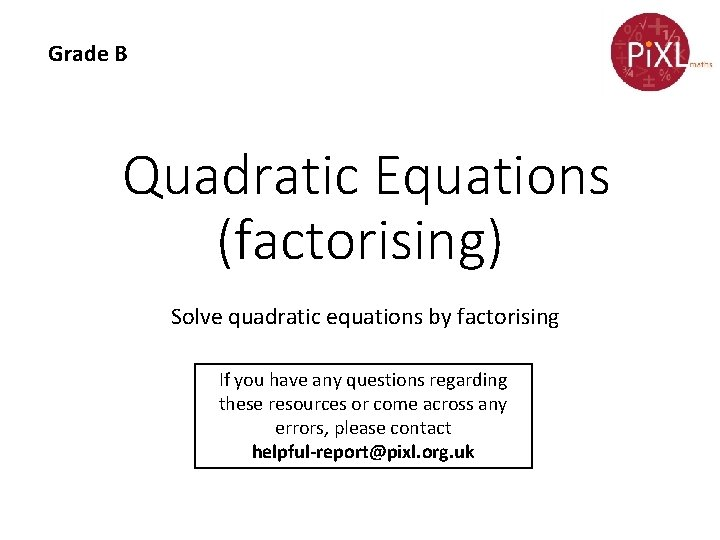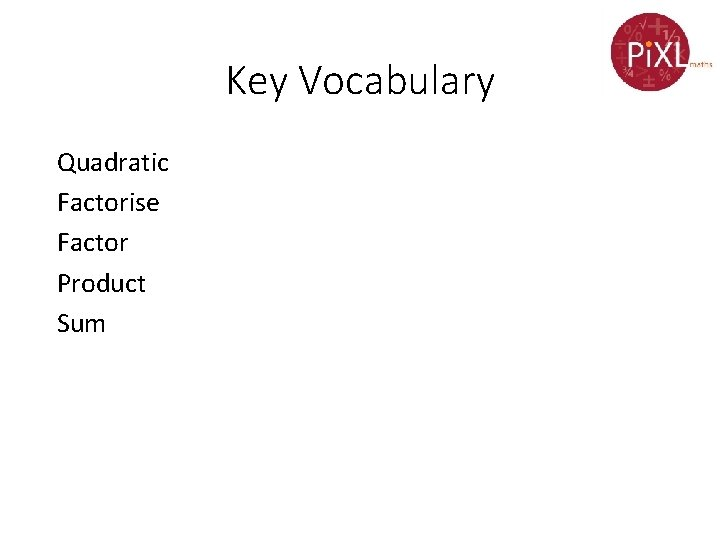Key Vocabulary Quadratic Factorise Factor Product SumHow to solve by factorising To solve by factorising we need two brackets of the form: (x+. . )=0 When we multiply two numbers together and the product is zero, then one of the numbers must have been zero. For example x 2 - 8 x +7 can be factorised as follows: ( x-7) ( x -1)How to solve by factorising So if x 2 - 8 x +7=0 then: ( x-7) ( x -1) =0 If the product of two numbers if zero then either (x-7)=0 or (x-1)=0 x-7=0 +7 +7 x=7 x-1=0 +1 +1 x=1 Therefore the solutions are x=7 and x=1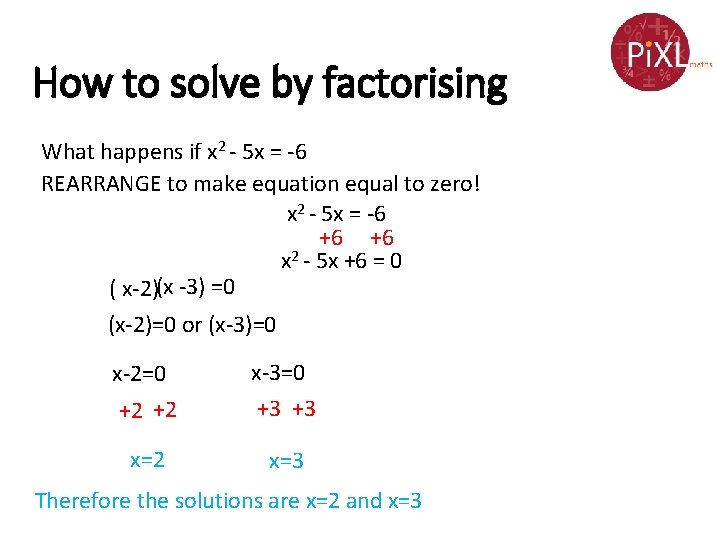How to solve by factorising What happens if x 2 - 5 x = -6 REARRANGE to make equation equal to zero! x 2 - 5 x = -6 +6 +6 x 2 - 5 x +6 = 0 ( x-2)(x -3) =0 (x-2)=0 or (x-3)=0 x-2=0 +2 +2 x-3=0 +3 +3 x=2 x=3 Therefore the solutions are x=2 and x=3Now you try Solve by factorising a)x(x+3)=0 b)x 2+ x -30 =0 c)x 2+ 18 x =-17 d) x 2 - 225 =0 e) 2 x(x-12) =0 f) 3 x 2+ 10 x +8 =0 g) 4 x 2 -24 x +27 =0 h) 16 x 2 -81 =0 i) 4 x 2 =6 x j) 3 x 2 -13 x =10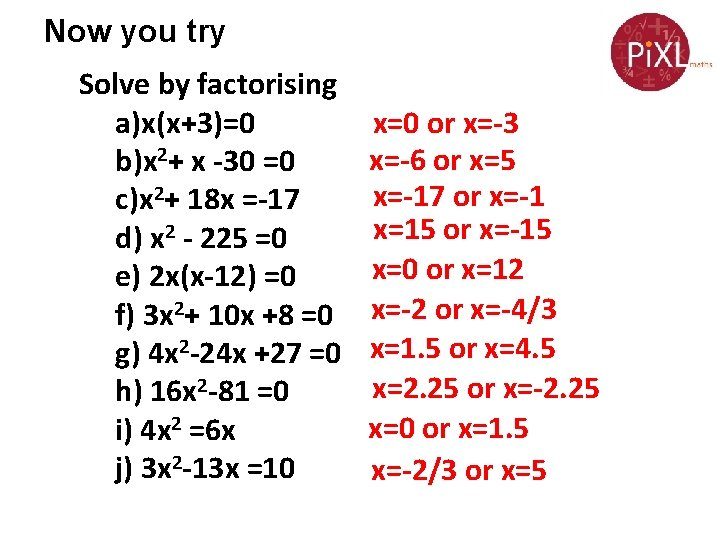Now you try Solve by factorising a)x(x+3)=0 b)x 2+ x -30 =0 c)x 2+ 18 x =-17 d) x 2 - 225 =0 e) 2 x(x-12) =0 f) 3 x 2+ 10 x +8 =0 g) 4 x 2 -24 x +27 =0 h) 16 x 2 -81 =0 i) 4 x 2 =6 x j) 3 x 2 -13 x =10 x=0 or x=-3 x=-6 or x=5 x=-17 or x=-1 x=15 or x=-15 x=0 or x=12 x=-2 or x=-4/3 x=1. 5 or x=4. 5 x=2. 25 or x=-2. 25 x=0 or x=1. 5 x=-2/3 or x=5Problem Solving A triangle has area 14 cm 2, by forming a quadratic equation find the value of x. Area of triangle: ½(base x perpendicular height) X-6 x-3 ½((x-6)(x-3))=14 X 2 (x-6)(x-3)=28 Need to make (x-6)(x-3) =x 2 -3 x -6 x +18 zero so have to expand brackets! 2 X -6 x - 3 x +18=28 Simplify 6 x and 3 x X 2 -9 x +18 =28Problem Solving X 2 -9 x +18 =28 We need to make this equal to zero to be able to factorise! x 2 -9 x +18 =28 -28 x 2 -9 x -10 =0 X-6 (x-10)(x+1)=0 X-3 (x-10)=0 +10 x=10 (x+1)=0 -1 -1 x=-1 Solutions are x=10 or x=-1. However in context of question -1 would mean the triangle wouldn’t exist!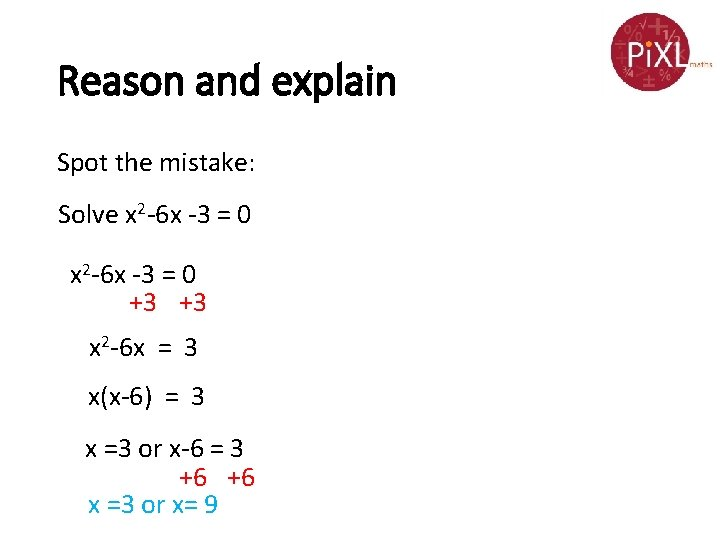Reason and explain Spot the mistake: Solve x 2 -6 x -3 = 0 +3 +3 x 2 -6 x = 3 x(x-6) = 3 x =3 or x-6 = 3 +6 +6 x =3 or x= 9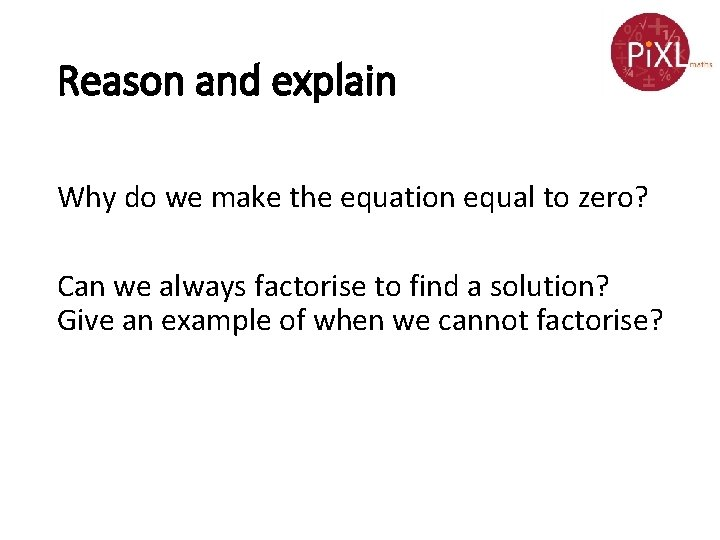Reason and explain Why do we make the equation equal to zero? Can we always factorise to find a solution? Give an example of when we cannot factorise?